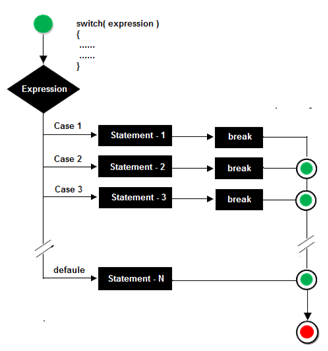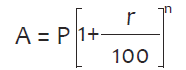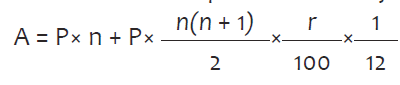Switch case Statement

A switch statement is used when there is requirement to check multiple condition in a program. It provides an easy way to branch to different parts of the code in program based on the value of an expression. It is a substitute for the large series of else if statements.

Syntax:switch(expression)
{
case  constant:
statement;
break;
case  constant:
statement;
break;
..
..
default:
statement;
}

Example Programs

Program 

Using the switch statement, write a menu driven program to calculate the maturity amount of a Bank Deposit.

The user is given the following options:

i. Term Deposit

ii. Recurring Deposit

For option (i) accept principal(P), rate of interest(r) and time period in years(n). Calculate and output the maturity amount(A) receivable using the formulaFor option (ii) accept Monthly Instalment (P), rate of interest(r) and time period in months(n). Calculate and output the maturity amount(A) receivable using the formulaFor an incorrect option, an appropriate error message should be displayed.